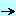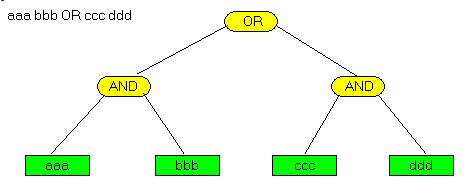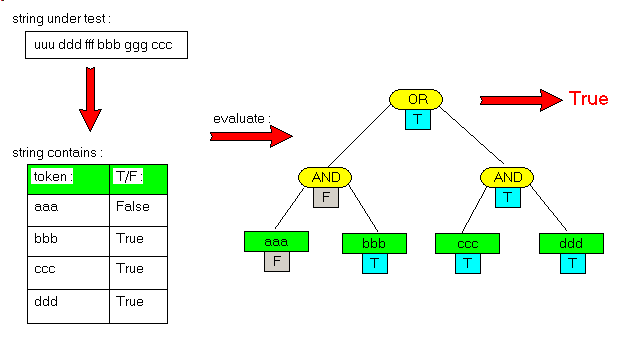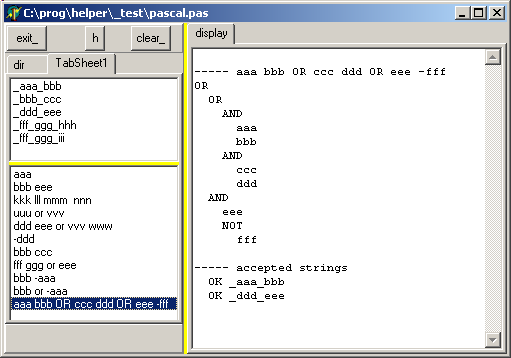Homepaperscolibri_utilitiesdelphi_string_filter

## Delphi String Filter - Felix John COLIBRI.

• abstract : a filter used for selecting pathes and file names based on a simple AST interpreter of a simple boolean string expression: "sale export OR 2018_01" accepts "2018_02_sales_exports.pdf" and rejects "2018_02_production.pdf"
• key words : string filter, parsing, Abstract Syntax Tree, interpreter
• software used : Windows XP Home, Delphi 6
• hardware used : Pentium 2.800Mhz, 512 M memory, 140 G hard disc
• scope : Delphi 1 to 2006, Turbo Delphi for Windows, Kylix
Delphi 5, 6, 7, 8 Delphi 2005, 2006, Delphi XE, Rad Studio
• level : Delphi developer
• plan :

## 1 - The String Filter purpose

The string filter implements a simple string filter allowing to accept or reject some string base on some substring content.

It was designed to filter pathes and directories. For instance, we can request

 export sales OR international contracts OR software -2017

meaning

 (*export* AND *sales*) OR     (*international* AND *contract*) OR     (*software AND NOT *-2017*)

and the following files would qualify

 export_sales_contracts.pdf international_purchase_contracts.ibx 2018_marketing.ppt soft_2028.pdf

and those would not

 sales_list.txt 2017_sofware_training.html

## 2 - The String Filter implementation

### 2.1 - The Filter Syntax

The syntax is the following
• the tokens are linked by implicit ANDs, the ORs have to be explicit
• case insensitive
• no "word only" filter
• no string delimiter, therefore the token cannot include spaces
Those choices are justified because the filter was created to analyze pathes and file names with minimal typing

### 2.2 - The string filter evaluation

In order to implement our filter, we use a simple AST interpreter:
• a binary tree is built where
• the terminal nodes are the litteral values
• the non terminal nodes are the operators, here AND, OR, NOT
• some string is entered.
• we first build a table containing as many entries as non-terminals
• we evaluate the value of the filter by walking in the binary tree
Let's take an example:
• our test expression is

 aaa bbb OR ccc ddd

• we build a table of the terminal values

 aaa bbb ccc ddd

• we built the binary AST tree:• we enter to following string

 uuu ddd fff bbb gggg ccc

• the array of the token contained in this string is

aaa False
bbb True
ccc True
ddd True

• and the evaluation of the tree isTo recap, the steps are the following
• the filter string is entered
• the tokens are saved in a token string array
• a simple parser builds the AST tree
Then, for each string to be evaluated
• we set the value of each token in the token array to True or False if the token is present in the string
• we call the evaluate function which will return whether the expression is true or not

### 2.3 - The Delphi AST tree

Basically we have to build a simple interpreter for a boolean expression. The BNF (IEBNF in our case: Indented Extended Backus Naur grammar notation) is:

 expression= term { OR term }     term= factor { AND factor }         factor= NOT factor | STRING

### 2.4 - The Delphi implementation

The litteral values are saved in a variable array:

 Type t_variable=          Record            m_variable_name: String;            m_contains: Boolean;          End;      c_variable_array=          Class(c_basic_object)            m_variable_array: Array Of t_variable;            m_variable_count: Integer;            Constructor create_variable_array(p_name: String);            Function f_add_variable(p_variable_name: String): Integer;            Function f_index_of(p_variable_name: String): Integer;            Procedure reset_values;            Procedure initialize_contains(p_string: String);            Procedure display_variable_array;          End; // c_variable_array

Then we define the Ast Nodes:

 Type c_node=          Class(c_basic_object)            m_c_variable_array_ref: c_variable_array;            Constructor create_node(p_name: String; p_c_variable_array_ref: c_variable_array);            Function f_evaluate_node: Boolean; Virtual; Abstract;            Procedure display_node; Virtual; Abstract;          End; // c_node      c_value_node=          Class(c_node)            // -- m_name : the litteral of the test            m_litteral_value: String; // alias of m_name            // -- the index in the variable array of the string to filter            m_variable_index: Integer;            Constructor create_value_node(p_name: String;                p_c_variable_array_ref: c_variable_array; p_variable_index: Integer);            Function f_evaluate_node: Boolean; Override;            Procedure display_node; Override;          End; // c_value_node      c_not_node=          Class(c_node)            m_c_not_node: c_node;            Constructor create_not_node(p_name: String; p_c_variable_array_ref: c_variable_array;                p_c_not_node: c_node);            Function f_evaluate_node: Boolean; Override;            Procedure display_node; Override;            Destructor Destroy; Override;          End; // c_not_node      c_binary_node=          Class(c_node)            m_c_left_node, m_c_right_node: c_node;            Constructor create_binary_node(p_name: String; p_c_variable_array_ref: c_variable_array;                p_c_left_node, p_c_right_node: c_node);            Procedure display_node; Override;            Destructor Destroy; Override;          End; // c_binary_node

The string filter contains both the variables and the root of the AST tree:

 Type c_string_filter=          Class(c_basic_object)            m_c_variable_array: c_variable_array;            m_c_root_node: c_node;            Constructor create_string_filter(p_name: String);            Procedure build_ast(p_filter: String);            Procedure display_ast;            Function f_filter_string(p_string: String): Boolean;            Destructor Destroy; Override;          End; // c_string_filter

The AST building is quite standard, aside from the read_symbol which is more intricate to account for the implicit AND :

 Procedure c_string_filter.build_ast(p_filter: String);     // -- "aaa bbb OR ccc OR ddd eee -fff   Type t_symbol_type= (e_unknown_symbol,       e_AND_symbol, e_OR_symbol, e_litteral_symbol, e_end_symbol);   Var l_index, l_length: integer;       l_symbol_string: String;       l_symbol_type: t_symbol_type;       l_is_term_start, l_read_next: Boolean;   Function _f_symbol_type: String;     Begin       Case l_symbol_type Of         e_unknown_symbol : Result:= 'unk';         e_AND_symbol : Result:= 'AND';         e_OR_symbol : Result:= 'OR ';         e_litteral_symbol: Result:= 'str';         e_end_symbol : Result:= 'end';       End;     End; // _f_symbol_type   Procedure _read_symbol;     Var l_string: String;     Begin       If l_is_term_start         Then Begin             If l_index>= l_length               Then Begin                   l_symbol_type:= e_end_symbol;                   l_symbol_string:= 'END';                   Exit;                 End;             l_symbol_string:= f_string_extract_non_blank(p_filter, l_index);             l_symbol_type:= e_litteral_symbol;             l_is_term_start:= False;             l_read_next:= True;           End         Else Begin             If l_read_next               Then Begin                   If l_index>= l_length                     Then Begin                         l_symbol_type:= e_end_symbol;                         l_symbol_string:= 'END';                         Exit;                       End;                   l_symbol_string:= f_string_extract_non_blank(p_filter, l_index);                   If SameText(l_symbol_string, 'OR')                     Then Begin                         l_is_term_start:= True;                         l_symbol_type:= e_OR_symbol;                         l_read_next:= True;                       End                     Else Begin                         l_symbol_type:= e_AND_symbol;                         l_read_next:= False;                       End;                 End               Else Begin                   l_symbol_type:= e_litteral_symbol  ;                   l_read_next:= true;                 End;           End;     End; // f__read_symbol   Procedure _display_error(p_error: String);     Begin       display_bug_stop('string_filter '+ p_error);     End; // _display_error   Function f_c_term: c_node;     Function f_c_factor: c_node;       Var l_variable_index: Integer;       Begin         Case l_symbol_type Of           e_litteral_symbol:               Begin                 If l_symbol_string= '-'                   Then Begin                       System.Delete(l_symbol_string, 1, 1);                       l_variable_index:= m_c_variable_array.f_add_variable(l_symbol_string);                       Result:= c_not_node.create_not_node('not', m_c_variable_array,                           c_value_node.create_value_node(l_symbol_string,                               m_c_variable_array, l_variable_index));                     End                   Else Begin                       l_variable_index:= m_c_variable_array.f_add_variable(l_symbol_string);                       Result:= c_value_node.create_value_node(l_symbol_string,                           m_c_variable_array, l_variable_index);                     End;                 _read_symbol;               End;           Else _display_error('string_litteral'); // NUMBER, NAME, (         End; // case       End; // f_c_factor     Begin // f_c_term       Result:= f_c_factor;       While l_symbol_type= e_AND_symbol Do       Begin         _read_symbol;         Result:= c_and_node.create_binary_node('and', Nil, Result, f_c_factor);       End; // WHILE {     End; // f_c_term   Begin // build_ast     l_index:= 1; l_length:= Length(p_filter);     l_is_term_start:= True;     _read_symbol;     m_c_root_node:= f_c_term;     While l_symbol_type= e_OR_symbol Do     Begin       _read_symbol;       m_c_root_node:= c_or_node.create_binary_node('or', m_c_variable_array,           m_c_root_node, f_c_term);     End;   End; // build_ast

Please note that the Pascal structure of the parser strictly parallels the IEBNF (expression, termn factor), which usually is the case for recursive descent parsers.

For each candidate string , we call the f_filter_string function:

 Function c_string_filter.f_filter_string(p_string: String): Boolean;   Var l_index: Integer;   Begin     With m_c_variable_array Do     Begin       reset_values;       initialize_contains(p_string);       // display_variable_array;     End;     Result:= m_c_root_node.f_evaluate_node;   End; // f_filter_string

and

• reset_values reinitalizes the value of the variable array:

 Procedure c_variable_array.reset_values;   Var l_variable_index: Integer;   Begin     For l_variable_index:= 0 To m_variable_count- 1 Do       m_variable_array[l_variable_index].m_contains:= False;   End; // reset_values

• we evaluate the value of each terminal of the candidate string:

 Procedure c_variable_array.initialize_contains(p_string: String);     // -- check if the string contains the litteral values   Var l_variable_index: Integer;       l_lowercase_string: String;   Begin     l_lowercase_string:= LowerCase(p_string);     For l_variable_index:= 0 To m_variable_count- 1 Do       With m_variable_array[l_variable_index] Do         m_contains:= Pos(m_variable_name, l_lowercase_string)> 0;   End; // initialize_contains

• finally we visit each tree of the AST node, evaluating the value of the node for this candidate string. We call the root node f_evaluate, and each node calls its own evaluation function:

 Function c_value_node.f_evaluate_node: Boolean;   Begin     Result:= m_c_variable_array_ref.m_variable_array[m_variable_index].m_contains;   End; // f_evaluate_node Function c_not_node.f_evaluate_node: Boolean;   Begin     Result:= Not m_c_not_node.f_evaluate_node;   End; // f_evaluate_node Function c_or_node.f_evaluate_node: Boolean;   Begin     Result:= m_c_left_node.f_evaluate_node Or m_c_right_node.f_evaluate_node   End; // evaluate_node Function c_and_node.f_evaluate_node: Boolean;   Begin     Result:= m_c_left_node.f_evaluate_node And m_c_right_node.f_evaluate_node;   End; // evaluate_node

### 2.5 - sample program

Wd want to test different filter on a list of candidate strings.

Our sample program will

• use a tListBox which will contain the candidate string
• have second tListBox with the different filters. Clicking on one filter will
• build the variable array
• build the AST tree
• for each candidate string, evaluate the value of the filter

Here is the test procedure

 Procedure TForm1.filter_listbox_Click(Sender: TObject);   Var l_c_string_filter: c_string_filter;   Procedure _build_filter;     Var l_filter: String;     Begin       With filter_listbox_ Do         l_filter:= Items[ItemIndex];       display_line;       display('----- '+ l_filter);       With l_c_string_filter Do       Begin         build_ast(l_filter);         display_ast;       End;     End; // _build_filter   Procedure _evaluate_strings;     Var l_list_index: Integer;         l_string: String;     Begin       display_line;       display('----- accepted strings');       With ListBox1 Do         For l_list_index:= 0 To COunt- 1 Do         Begin           l_string:= Items[l_list_index];           With l_c_string_filter Do             If f_filter_string(l_string)               Then display('  OK '+ l_string)         End;     End; // _evaluate_strings   Begin // filter_listbox_Click     clear_display;     l_c_string_filter:= c_string_filter.create_string_filter('');     _build_filter;     _evaluate_strings;     l_c_string_filter.Free;   End; // filter_listbox_Click

and the result is:Here are the source code files:
The .ZIP file(s) contain:
• the main program (.DPR, .DOF, .RES), the main form (.PAS, .DFM), and any other auxiliary form
• any .TXT for parameters, samples, test data
• all units (.PAS) for units
Those .ZIP
• are self-contained: you will not need any other product (unless expressly mentioned).
• for Delphi 6 projects, can be used from any folder (the pathes are RELATIVE)
• will not modify your PC in any way beyond the path where you placed the .ZIP (no registry changes, no path creation etc).
To use the .ZIP:
• create or select any folder of your choice
• using Delphi, compile and execute
To remove the .ZIP simply delete the folder.

The Pascal code uses the Alsacian notation, which prefixes identifier by program area: K_onstant, T_ype, G_lobal, L_ocal, P_arametre, F_unction, C_lass etc. This notation is presented in the Alsacian Notation paper.

As usual:

• we welcome any comment, criticism, enhancement, other sources or reference suggestion. Just send an e-mail to fcolibri@felix-colibri.com.
• or more simply, enter your (anonymous or with your e-mail if you want an answer) comments below and clic the "send" button
 Name : E-mail : Comments * :

## 4 - References

We published another paper about expression evaluations:

## 5 - The author

Felix John COLIBRI works at the Pascal Institute. Starting with Pascal in 1979, he then became involved with Object Oriented Programming, Delphi, Sql, Tcp/Ip, Html, UML. Currently, he is mainly active in the area of custom software development (new projects, maintenance, audits, BDE migration, Delphi Xe_n migrations, refactoring), Delphi Consulting and Delph training. His web site features tutorials, technical papers about programming with full downloadable source code, and the description and calendar of forthcoming Delphi, FireBird, Tcp/IP, Web Services, OOP  /  UML, Design Patterns, Unit Testing training sessions.
 Created: feb-18. Last updated: dec-2019 - 103 articles, 239 .ZIP sources, 1292 figures Contact : Felix COLIBRI - Phone: (33)1.42.83.69.36 / 06.87.88.23.91 - email:fcolibri@felix-colibri.com Copyright © Felix J. Colibri   http://www.felix-colibri.com 2004 - 2019. All rigths reserved Back:    Home  Papers  Training  Delphi developments  Links  Download### Felix J COLIBRI

+ Home
+ articles_with_sources
+ database
+ web_internet_sockets
+ rest_services
+ oop_components
+ uml_design_patterns
+ debug_and_test
+ graphic
+ controls
+ colibri_utilities
– delphi_net_bdsproj
– dccil_bat_generator
– coliget_search_engine
– dfm_parser
– dfm_binary_to_text
– component_to_code
– exe_dll_pe_explorer
– dll_process_viewer
– the_alsacian_notation
– html_help_viewer
– cooking_the_code
– events_record_playback
– colibri_hiragana_quiz
– directory_favorite_list
+ colibri_helpers
+ delphi
+ IDE
+ firemonkey
+ compilers
+ vcl